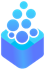# OpenDreamKit

## A project funded by the Horizon 2020

European Research Infrastructures Work Program

# Progress report for Kaiserslautern

Wolfram Decker

Reporting period from March 2017 to January 2018

• TODO Michael

## Hiring

• Daniel Schultz, postdoc 100%
• 1 July 2017 – 30 Sept. 2019
• Research Associate at Penn State University
• Rémi Imbach, postdoc 100%
• 1 June 2017 – 31 March 2018

• Designer and developer of certified numerical solver subdiv_solver at INRIA Nancy - Grand Est

• Numerical methods for solving systems of polynomials

• Computational algebraic geometry

## Work in progress

### Parallel Sparse Polynomial Arithmetic in Singular

The goal of the project is to implement in Singular fast parallel arithmetic for multivariate polynomials defined over either the integers ($\mathbb{Z}$), the rational numbers ($\mathbb{Q}$), or the integers modulo a prime ($\mathbb{Z}/n\mathbb{Z}$). The implementation should include the basic operations (addition, subtraction, multiplication, exact division, and powering), the various kinds of inexact division, and a computation of the greatest common divisor (GCD) of two polynomials. These operations should also be parallelized.

The main library in Singular for arithemtic is Factory, which makes use of FLINT for polynomials rings. Because the work is being done to a library available in Singular (FLINT), it is directly available to other projects with the OpenDreamKit progect. The repository is located at https://github.com/wbhart/flint2/tree/fmpz_mpoly.

The \emph{mpoly} module for dealing with multivariate exponents has been rewritten to allow for arbitrary packings of the exponents into fields within one machine word as well as over multiple machine words, a strategy employed by singular. This means that small exponents as well as large multi-precision exponents can be dealt with efficiently. However, only the basic operations (addition, subtraction, multiplication, exact division, and powering) are allowed to work on multi-precision exponents. For multiplication and division of polynomials, the algorithms are further divided into a “sparse” case and a “semi-sparse” case, where sparse data stuctures and algorithms are sill employed, but traditional sparse alrorithms are not optimal.

So far all of the various kinds of division over $\mathbb{Z}$ and $\mathbb{Z}/n\mathbb{Z}$ (including quasi-division over $\mathbb{Z}$) are implemented. For GCD computations, only the very beginnings are implemented: For the coefficient field $\mathbb{Z}$, an algorithm based on polynomial remainder sequences (PRS) is implemented for GCD, discriminant, and resultant computations. This algorithm is not particularly efficient on large problems. For the coefficient field $\mathbb{Z}/n\mathbb{Z}$, Brown’s modular GCD algorithm is implemented but not optimized. The only operation that is parallelized at this point is the sparse polynomial multiplication, but the implementation scales well to multiple worker threads while still being fast with only one worker thread.

We have extensively benchmarked our parallel sparse multiplication algorithm against all open and close source libraries we can find. On real world problems our timings are world-class, if not world-beating.

Semi-sparse multiplication still needs to be parallelized along with semi-sparse and sparse division. Parallelizing the division routines is going to be a challenging task. For GCD computations over $\mathbb{Z}/n\mathbb{Z}$, we further need to optimize and parallelize Brown’s modular GCD in the dense/semi-sparse case and improve and parallelize the existing implementation of Zippel’s algorithm in Factory/Singular. For GCD computations over $\mathbb{Z}$, we need to combine the PRS algorithm, a modular approach based on GCD computations over $\mathbb{Z}/n\mathbb{Z}$, as well as a new sparse interpolation algorithm due to Daniel Roche. Polynomials over $\mathbb{Q}$ will be implemented as the quotient of polynomial and a common denominator, so the operations over $\mathbb{Q}$ will be simple wrappers around the corresponding operations over $\mathbb{Z}$.

This project is coming along nicely, and the deliverables currently on time, if not slightly ahead of schedule.

### Parallel root clustering in Singular

As mentioned in the proposal, parallelizing polynomial arithmetic is only one part of our work on fine-grained parallelism in Singular. In addition to the above deliverable, we have been able to take advantage of a great opportunity to implement a new algorithm for clustering the roots of a univariate polynomial whose coefficients are complex algebraic numbers, described in becker2016. The main advantages of this algorithm compared to state-of-the-art complex root isolators are:

• its ability to find clusters of roots up to a size $\epsilon$, \emph{i.e.} it finds complex disks of size less than $\epsilon$ containing roots, and for each disk the number of roots counted with multiplicity it contains
• its ability to work locally, \emph{i.e.} it doesn’t search for all the roots, but only for the ones lying in a given initial complex disk
• its ability to work with inexact input polynomials: the coefficients of the input polynomial are considered as black-boxes delivering as many exact bits as desired.

The current version of the implementation is publicly released under license LGPL-2.1, and is available at https://github.com/rimbach/Ccluster. We will now focus on three tasks:

• parallelizing the implementation; the algorithm uses a classical subdivision approach that is naturally parallel. However, we will also experiment with parallelizing lower level operations, particularly polynomial arithmetic.
• delivering user-friendly interfaces for Singular and/or Julia
• delivering a stand-alone version with inputs/outputs similar to the state-of- the-art complex root isolator so that it can easily be plugged in higher level computing libraries, for instance CGAL.

</section>

<